# OOF: Finite Element Analysis of Microstructures

Background Material: Basic Anisotropic ThermoelasticityNext: Plane Stress and Plane Up: The Element Types Previous: Coordinate Conventions   Contents

# Background Material: Basic Anisotropic Thermoelasticity

In the current version of OOF, all elements are triangles consisting of three nodes located at the triangle vertices. The nodes may be located at the boundary of the mesh, or they can be shared with other elements. The elements share information with each other (i.e., how much the corners have been displaced) through their nodes.

The current version of OOF is thermoelastic, and so the pertinent field quantities are the displacement (vector) field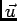and the uniform temperature change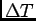. The displacement field imparts a strain (tensor) field through the rotation-invariant values of spatial derivatives of the displacement field: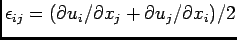-which is a long-winded way of saying the nodal displacements impose a strain,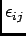, on the elements through a predictable formula.

The temperature is given as a difference. This difference is relative to the temperature at which the initial mesh is stress free. Each element undergoes a uniform strain,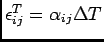, in the stress free state (i.e., if the nodes are completely unrestrained or the stress tensor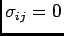). The second rank tensor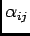is the (symmetric) thermal expansion tensor. It has additional symmetries according to the point group of the underlying material. See Nye  for a lucid discussion of how material symmetry is expressed in tensors representing linear constitutive behavior.

The stress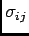is a second rank tensor which represents the the total force transmitted in the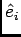-direction through a planar region with its normal parallel to the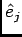-direction in the limit that the area of the region shrinks to a point. Torque balance requires that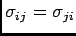in linear elasticity.

In linear elasticity, the stress is related to the strains implied by the displacement field and the stress-free strain4.1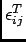through the linear relation: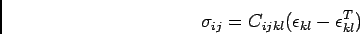The fourth-rank tensor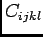is the stiffness tensor. The symmetry of bothand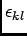imply that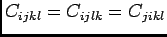, so it can have at most 36 independent components. An additional requirement, that the elastic energy density be frame invariant, imposes the symmetry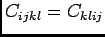and reduces the number of independent components to 21.

It is convenient to take advantage of the symmetries to represent the stiffness tensor as a symmetric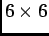matrix. This can lead to silly mistakes since the matrix does not rotate in the usual straight-forward way. Fortunately, OOF handles the rotations for you (see Section 4.3). To convert the fourth-rank tensor into a two dimensional matrix, we adopt the convention in Nye  and replace the six distinct pairs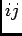with a single integer as follows:
 Four Index Tensor Indices11 22 33 23 or 32 31 or 13 12 or 21 Two Index Matrix Indices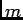1 2 3 4 5 6
For example, the tensor components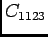,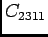,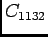, and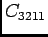, which must all be equal by symmetry, are all represented by the matrix element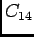.

In OOF, the stiffness components are entered using the matrix notation. The symmetry of each particular material places additional constraints on the stiffness matrices. These additional symmetries are illustrated in Figures 4.2, 4.3, 4.4, and 4.5 (following Nye ).Next: Plane Stress and Plane Up: The Element Types Previous: Coordinate Conventions   Contents
/* Send mail to the OOF Team *//* Go to the OOF Home Page */